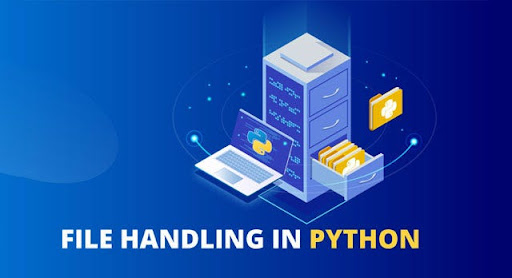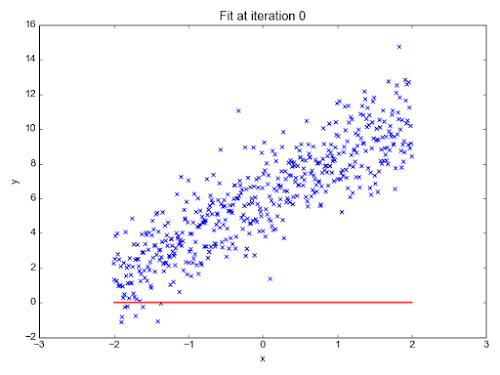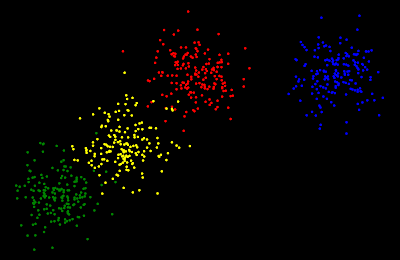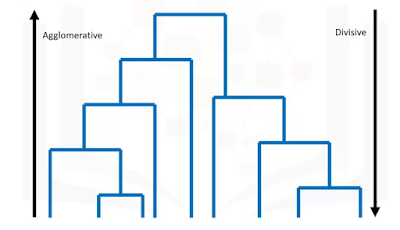## Posts

Showing posts from 2020

### Pose Estimation || Application of Computer Vision and Deep LearningWhat is Pose Estimation?Pose Estimation is one of the recent applications of Deep Learning and Computer Vision. In pose estimation we try to predict the pose of a person, it means representing an orientation of a person in graphical format. While reading out the intro it might look a bit complex but actually, it is not. We implement the idea by considering each joint of our body as a coordinate for our graphical representation. The bones which connect two joints are shown as a simple line.To make a posture simple and easy to visualize we do not represent each and every bone of a human body. Instead, we represent Hands, Legs, Shoulder and Face of a person.

BackgroundPreviously, one of the famous application of computer vision was face detection and face recognition. We also used to apply computer vision for detecting whether a person is present in an image or not. If the presence is detected then we can highlight the area where it is present. So, after making some advancements and a bit…

### File Handling with Python || Python programming tutorialIn File Handling, we will be working on a file in our computer and doing various operations on it. Check out my previous blog on Python programming if you want to learn from scratch.
Let us say we have a file name poem.txt and we need to perform various operations on that file. Reading the whole file and printing itFirst, we need to open that text file in our python program using a function open().The open() function will take two arguments filename and set our intentions.Now, you must be wondering about the second argument i.e. we need to specify our purpose of using that file Read, Write and AppendAbove all operations can be specified by with different alphabets:Read - 'r'Write - 'w'Append - 'a'Now we will implement programs with this knowledge
Program -  f = open("poem.txt", "r") f.read() Counting Number of Lines in our fileWe will divide this process into various steps : To read a single line of a file we can use readline method
Program -
f = op…

### Getting Started with your first Machine Learning Algorithm - Linear Regression || First step towards MLLinear Regression is a Supervised Learning Algorithm. This is probably the first algorithm taught when you start learning ML. Now as a beginner you must be wondering "What is a Supervised Learning??". So machine learning can be divided mainly into 3 types: Supervised LearningUnsupervised LearningReinforcement LearningSupervised Learning is a technique in which we use a labelled dataset to train a model. In short, you can say there is a supervisor who will tell whether the predictions model is making is correct or not. This supervisor decides about based on the labels provided in the datasets. Labels are the correct values for a particular training example. We will deal later with the other two techniques of ML.
In supervised learning, we can classify problems into 2 different categories: Regression - problems in which we need to predict continuous value as an output.Classification - where we need to classify the training examples in a particular category. We plot all the trainin…

### Implementing Hierarchical Clustering - In Python Programming languageIntroduction to Hierarchical Clustering
Unsupervised learning is a type of Machine learning in which we use unlabeled data and we try to find a pattern among the data. Clustering algorithms falls under the category of unsupervised learning. In these algorithms, we try to make different clusters among the data. Hierarchical Clustering algorithms build a hierarchy of clusters where each node is a cluster consisting of the clusters of its children node.

Check out my blog on Hierarchical Clustering - An Unsupervised learning Algorithm to learn more about it.
Implementing it with Python and SciKit-Learn
We will use the python programming language for its implementation. In python language, we will be using SciKit-Learn library. So let's start with implementation - We will be using our own dataset which will be generated by us.

1. Importing necessary Libraries -

To create a machine learning model we will use SciKit-Learn (sklearn) library and to create our dataset we will use SciPy library.
…

### Hierarchical Clustering - An Unsupervised Learning AlgorithmIntroduction
Unsupervised learning is a type of Machine learning in which we use unlabeled data and we try to find a pattern among the data. Clustering algorithms falls under the category of unsupervised learning. In these algorithms, we try to make different clusters among the data. Hierarchical Clustering algorithms build a hierarchy of clusters where each node is a cluster consisting of the clusters of its children node.
There are various strategies in Hierarchical Clustering such as : DivisiveAgglomerative
This type of diagram is called Dendrogram. Divisive - It is a Top-down approach. So we start with all observations in a large cluster and break it down into smaller ones. Agglomerative - It is the opposite of Divisive as it is a Bottom-Up approach. Here, each observation starts in its cluster and pairs of the cluster are merged as they move up the hierarchy.  (Generally, Agglomerative is used more as compared to Divisive among Data Sci…

### What is Python ?? || Introduction to Python programmingWhat is Programming?👀💻
In simple language, we can say programming is a technique through which we instruct the computer to perform various tasks.
To instruct computer we need to communicate with a computer and for communication, we need a language as a medium. So programming language serves as a medium of communication between the user and a computer. But, as we know computer only understand binary language and it is practically not possible for us to use binary language with only 0s and 1s.
To solve this problem we need some translator called the compiler. The compiler will compile the code and generate a binary file of it which will be understood by the computer. So this is a complete process of how a computer can be programmed to perform a certain task. Besides compiler there is also an Interpreter.
What is Python?🐍
Python is a high level programming language. Designed by Guido Van Rossum. Now a days python is majorly used for DataScience field i.e. Machine Learning, Artificial Inte…

### Reinforcement Learning - An Introduction || Getting started with RLIntro to Reinforcement LearningPhoto by James Pond on Unsplash
“Success in creating AI would be the biggest event in human history. Unfortunately, it might also be the last, unless we learn how to avoid the risks.” — Stephen Hawking
Reinforcement Learning is a category of Machine learning which is again a subset of an Artificial Intelligence (AI). We all have experienced AI somehow in our day to day life. The implementation of AI has a vast range of applications in the domain of Education, Finance, Medical, Agriculture, Electronics, Transport, etc.
Now, moving on to machine learning which is a subset of AI. Machine learning is the field of study that gives the computers an ability to learn without being explicitly programmed.

Moving towards Reinforcement LearningThe idea of reinforcement learning is inspired by an idea about how the human brain works. So there are a lot of analogies between them. Reinforcement learning (RL) is trial and error based method in which an agent will perform a…